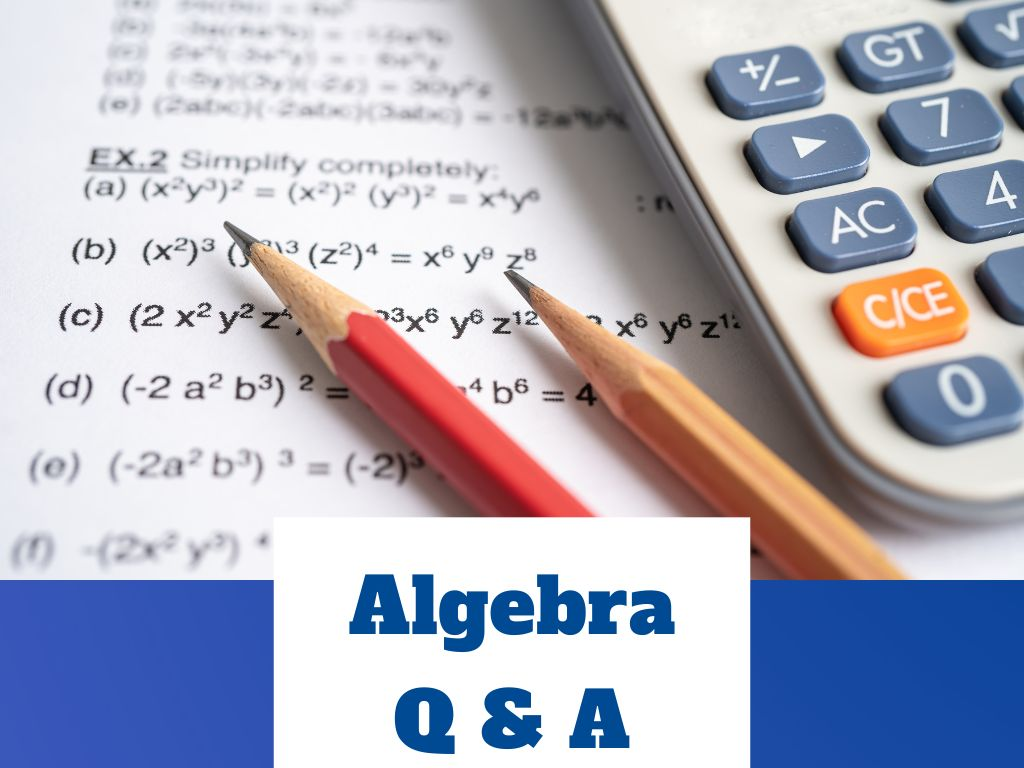## Algebra Q & A

Q 1) Are polynomials linear? Ans. – Yes, polynomials are linear and called linear polynomials. The standard form of a linear polynomial is ax + b where, a ≠ 0. Example – 2x + 3, x – 5, 7x, etc. Q 2) Are polynomials closed under division? Ans. – No, polynomials are not closed under …